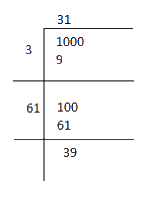# Ex.6.4 Q8 Squares and Square Roots - NCERT Maths Class 8

Go back to  'Ex.6.4'

## Question

A gardener has $$1000$$ plants. He wants to plant these in such a way that the number of rows and the number of columns remain same. Find the minimum number of plants he needs more for this.

If number of rows and number of columns are equal then number of plants has to be a perfect square

Video Solution
Squares And Square Roots
Ex 6.4 | Question 8

## Text Solution

What is known?

Number of plants.

What is unknown?

Minimum number of more plants to make the number of rows and number of columns same.

Reasoning:

If number of rows and number of column are equal then number of palant has to be a prefect square.

Steps:

Number of plants $$= 1000$$

The square root of $$1000$$ can be calculated by long division method.The remainder is $$39.$$

It shows that $${31^2} < 1000$$

The square of $$32$$ is $$1024$$

Hence number to be added to $$1000$$ to make it perfect square

.\begin{align} &= {32^2} - 1000\\ &= 1024 - 1000\\ &= 24 \end{align}

Thus, the required number of plants $$=24$$

Learn from the best math teachers and top your exams

• Live one on one classroom and doubt clearing
• Practice worksheets in and after class for conceptual clarity
• Personalized curriculum to keep up with school